> Example: Create a guided loft with scalar variation

Example: Create a guided loft with scalar variation
Scaling is applied to the profiles with respect to the spine. When a profile is calculated for a certain position of the spine, this position is the center for the scaling. The correlation of this position and the calculated profile depends on the original attachment points. An attachment point is the intersection of the profile's plane and the spine.
For non-planar or non-orthogonal profiles where the attachment point position is not clearly defined, the result may be unexpected.
Spine (orange) and profile (cyan) for a guided loft with scaling: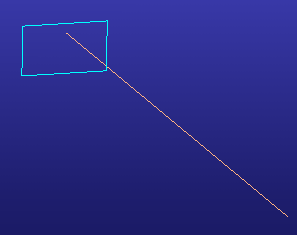Guided loft with Scalar: Constant. A constant scale is applied along the spine: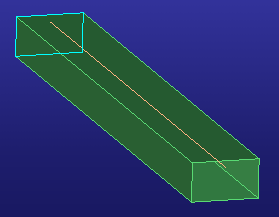Guided loft with Scalar: Linear. Specify a start and end value: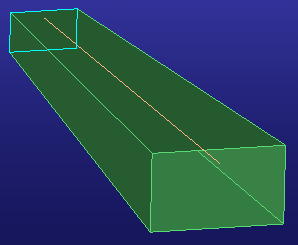Guided loft with Scalar: Parabolic. Define three values: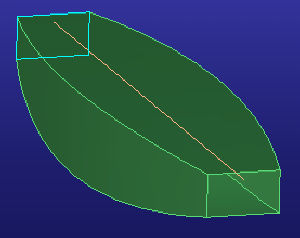Guided loft with Scalar: Two Values: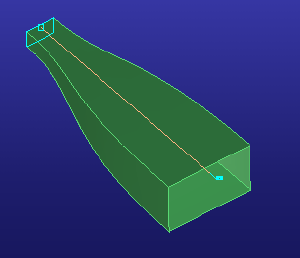Two Values
Define two scale values and two weights (Start and End). The larger the weight, the longer the scale value is kept before the other value is used for sweeping the profile along the spine.
A weight of 0 results in linear scaling.
Guided loft with Scalar: Three Values: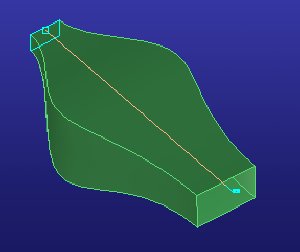Three Values
Define three scale values and two weights. The midpoint can be shifted forward or backward on the spine in order to change the shape of the surface. Change the location of the midpoint by entering a value between 0.1 and 0.9 in Mid Par.
A weight of 0 results in parabolic scaling.
Guided loft with Scalar: 2D Curve: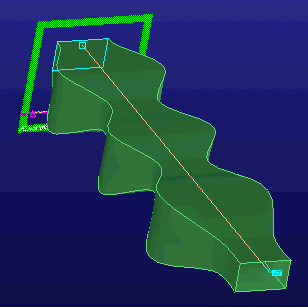2D Curve
To use the 2D Curve function, you must have a spline curve created on a workplane. The U axis of the workplane is mapped to the spline curve. The values of the spline along the V axis are used as the scaling function's values.
Select the 2D curve on the workplane. This curve is used to scale the profile.
Select the Origin by clicking a 2D point on the workplane. The origin has an influence on the V value of the 2D curve. If the distance between the start point of the 2D curve and the origin is 1 (the origin can be anywhere along the U axis), the scaling value at the start of the spine is 1. The distance between the 2D curve and the origin is measured in mm. 1mm = factor of 1.
The distance in the direction of the V axis is interpreted as the "value" of the function at the U position (a relative position on the spine between the start and end of the spine).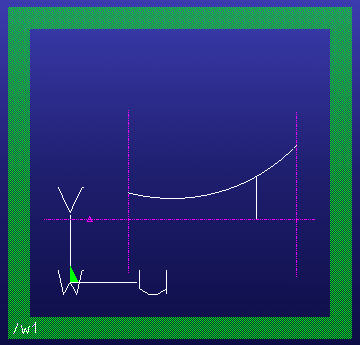The Origin is shown by the construction point. The 2D curve has a start position at the first construction line and an end position at the second construction line. The vertical line shows the distance - in the V direction - between the U position of the spine and the origin.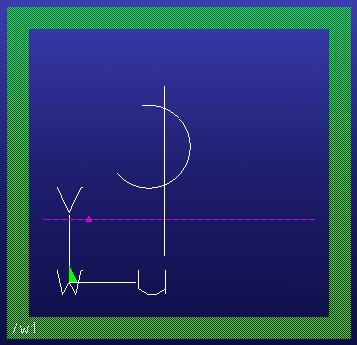A 2D curve that contains two V values (on the vertical line) for one U value (relative position on the spine) cannot be used as a function. If a vertical line (parallel to the V axis) intersects the curve more than once, the curve cannot be used for scaling.
The Origin is shown by the construction point. The V direction intersects the 2D curve twice.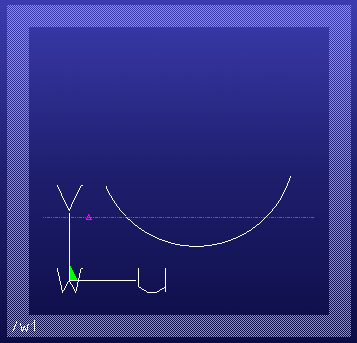The 2D curve cannot be negative, or smaller than the V value of the origin because the distance would be negative and the surface would be self-intersecting.
The Origin is shown by the construction point. The 2D curve and the function become negative with respect to the V value of the Origin.